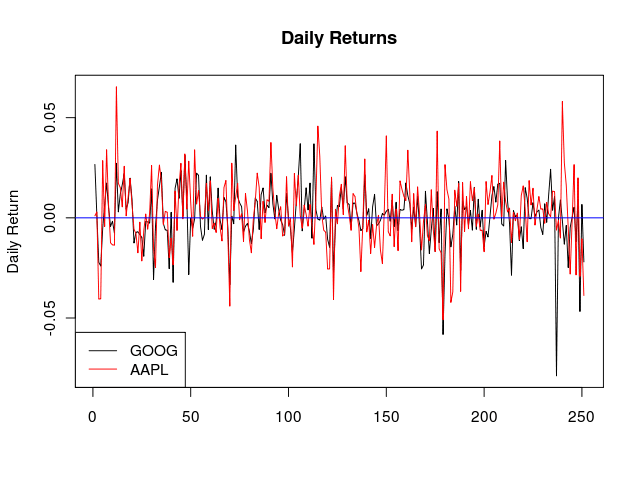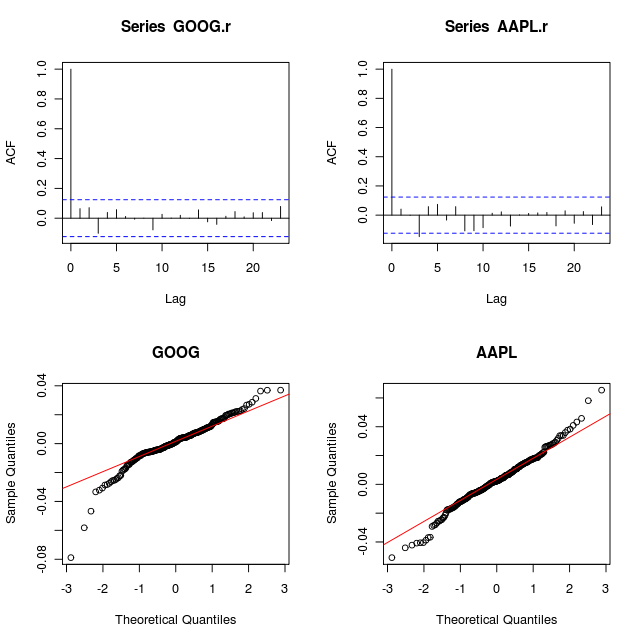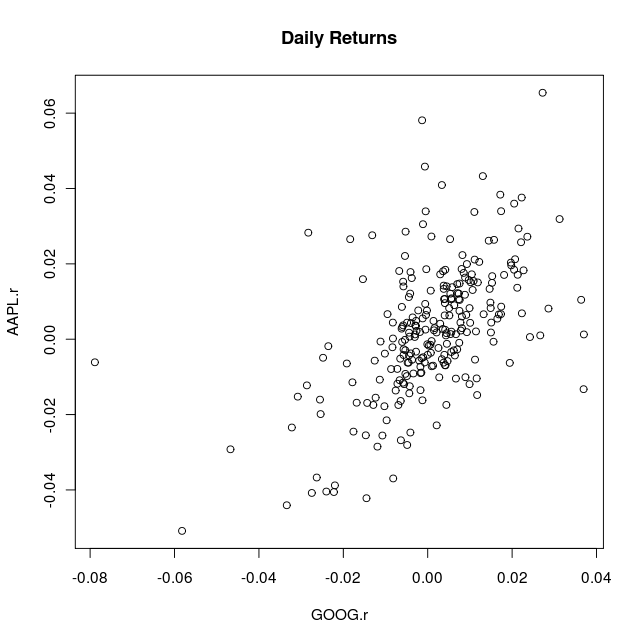Want to share your content on R-bloggers? click here if you have a blog, or here if you don't.

I find options fascinating because they deal with the abstract ideas of volatility and correlation, both of which are unobservable and can often seem like wild animal spirits (take the current stock market as an example). Understanding these subtle concepts is never easy, but it is essential in pricing some of the more exotic options which involve multiple underlying stocks. To set the scene, let’s pretend that your neighbor wants to make a bet with you where he will pay you \$100 if Google (GOOG) and Apple (APPL) are above 500 and 240 respectively after 1 year, but you have to pay him \$25 today. How would we determine if \$25 is a good deal or not?

# The Model

The first things we need to do is to define the dynamics for all the tradable assets. We have at our disposal a money market account, as well as the stocks of Google and Apple (it is not a coincidence that I picked companies that don’t pay dividends). Below describes how we will model the dynamics of these assets.$dS_t^{(0)} = S_t^{(0)} r_t dt \ dS_t^{(1)} = S_t^{(1)} (mu_t^{(1)} dt + sigma_t^{(1)} dW_t^{(1)}) \ dS_t^{(2)} = S_t^{(2)} (mu_t^{(2)} dt + sigma_t^{(2)} dW_t^{(2)}) \ text{where } dW_t^{(1)}dW_t^{(2)}=rho dt$

In this model,$S_t^{(0)}$ is the price of a share in the money market account, and$S_t^{(1)}$ and$S_t^{(2)}$ are the stock prices. Notice that we have correlated the Brownian Motions$W_t^{(1)}, : W_t^{(2)}$ such that the stock prices will tend to move up and down together just like in the real world. To make this model easier to work with, we will first convert the correlated Brownian Motions into independent Brownian Motions$B_t^{(1)}, : B_t^{(2)}$ by defining the following.$dW_t^{(1)}=dB_t^{(1)} \ dW_t^{(2)}=rho dB_t^{(1)} + sqrt{1-rho^2}dB_t^{(2)} \ text{where } dB_t^{(1)}dB_t^{(2)}=0$

By doing this, we can rewrite the ‘real world’ dynamics as$dS_t^{(1)} = S_t^{(1)} (mu_t^{(1)} dt + sigma_t^{(1)} dB_t^{(1)}) \ dS_t^{(2)} = S_t^{(2)} (mu_t^{(2)} dt + sigma_t^{(2)} (rho dB_t^{(1)} + sqrt{1-rho^2}dB_t^{(2)}))$

# Calibration

Writing down a bunch of stochastic differential equations was pretty mechanical, but now comes the artsy part of modeling. The first thing we need is some stock price data, which can easily be downloaded from Yahoo Finance. This data gives us the stock price over discreet time intervals (I picked daily close prices). To use this data, lets define the discreet return$r_n$ as the solution to the following$S_{n+1}=S_n e^{r_n}$, in which case we can write$r_n=log left( frac{S_{n+1}}{S_n} right)$. By assuming the drift and volatility terms are constants, we can use our model of the stock price dynamics to write the discreet returns as the following.$r_n^{(1)}=mu^{(1)} delta t + sigma^{(1)} sqrt{delta t} z_1 \ r_n^{(2)}=mu^{(2)} delta t + sigma^{(2)} sqrt{delta t} ( rho z_1 + sqrt{1-rho^2} z_2) \ text{where } z_1, z_2 sim iid : N(0,1)$

Where$delta t$ is the discreet time interval. By doing this, we can show that the discreet returns are distributed iid normal.$r_n^{(1)} sim iid : N(mu^{(1)} delta t, : sigma^{(1)} sqrt{delta t}) \ r_n^{(2)} sim iid : N(mu^{(2)} delta t, : sigma^{(2)} sqrt{delta t})$

We can use the iid normal distribution to calibrate all the parameters.$sigma^{(i)}$ are simply the sample standard deviations of the discreet returns divided by$sqrt{delta t}$,$rho$ is the sample correlation of the discreet returns, and$mu^{(i)}$ are the sample means of the discreet returns divided by$delta t$ (although I should note that we don’t actually need to know$mu^{(i)}$ to price this option).

# The Data

Now that we have the math worked out for calibrating the model, let’s take a look at some real data and see if the data agrees with our assumptions. First we need to read the data into R. The data I used can be found here. (I used R’s scan function since I’m lazy).

```## Scan in close prices
GOOG <- scan()
AAPL <- scan()```

Let’s take a look at the time series of stock prices.

```## regular stock price chart
par(mfrow=c(2,1))
plot(GOOG, type="l", main="GOOG closing Price")
plot(AAPL, type="l", main="AAPL closing Price")```Now calculate and plot the daily returns.

```## convert to returns
n <- length(GOOG)
GOOG.r <- log(GOOG[2:n]/GOOG[1:(n-1)])
AAPL.r <- log(AAPL[2:n]/AAPL[1:(n-1)])

## plot returns
matplot(cbind(GOOG.r, AAPL.r), type="l", lty=1, main="Daily Returns", ylab="Daily Return")
abline(h=0, col="blue")
legend("bottomleft", c("GOOG", "AAPL"), lty=1, col=c(1,2))```To check the iid normal assumption, we should do an autocorrelation plot and a QQ normal plot.

```## autocorrelation plot
par(mfrow=c(2,2))
acf(GOOG.r)
acf(AAPL.r)

## QQ normal plots
qqnorm(GOOG.r, main="GOOG")
qqline(GOOG.r, col="red")
qqnorm(AAPL.r, main="AAPL")
qqline(AAPL.r, col="red")```Lastly, lets also take a look at the joint distribution of the returns.

```## pairs plot
plot(GOOG.r, AAPL.r, main="Daily Returns")```The ACF plot suggests that the daily returns are iid, but they certainly have heavier tails than what would be produced by a normal distribution. In order to keep the model simple, we’ll assume normality of the returns holds, and give ourselves a ‘buffer’ when we calculate the fair price. Finally, time for the calibration!

``` GOOG vol: 0.2262006 AAPL vol: 0.2756421 Rho: 0.5413732 ```

```## calculate annualized vol
GOOG.vol <- sd(GOOG.r)/sqrt(1/252)
AAPL.vol <- sd(AAPL.r)/sqrt(1/252)

## calculate rho
rho <- cor(GOOG.r, AAPL.r)

cat("GOOG vol:", GOOG.vol, "n")
cat("AAPL vol:", AAPL.vol, "n")
cat("Rho:", rho, "n")```

# Price Using Simulation

We are now at the point where we can price this option. As usual, the price of the option is the expected discounted payoff under the risk neutral measure. This particular option can be priced as

```trials <- 10000     # simulation trials
T <- 1              # time until expiration (years)
m <- 200            # subintervals
dt <- T/m           # time per subinterval (years)

## set interest rate model parameters
k <- 0.4
theta <- 0.05
beta <- 0.03

## set strikes
K1 <- 500
K2 <- 240

## create storage vectors
s1 <- rep(0,m+1)       # stock price path 1
s2 <- rep(0,m+1)       # stock price path 2
r <- rep(0,m+1)        # interest rate
c <- rep(0, trials)    # payoff

## set initial stock prices and interest rate
s1 <- 500
s2 <- 240
r  <- 0.01

## begin simulation
for(j in 1:trials){
for(i in 2:(m+1)){
z1 <- rnorm(1,0,1)
z2 <- rnorm(1,0,1)
z3 <- rnorm(1,0,1)

ds1 <- s1[i-1]*(r[i-1]*dt + GOOG.vol*sqrt(dt)*z1)
ds2 <- s2[i-1]*(r[i-1]*dt + AAPL.vol*sqrt(dt)*(rho*z1 + sqrt(1-rho^2)*z2))
dr  <- k*(theta - r[i-1])*dt + beta*sqrt(dt)*z3

s1[i] <- s1[i-1] +  ds1
s2[i] <- s2[i-1] +  ds2
r[i]  <- r[i-1]  +  dr
}
ss <- sum(r[2:(m+1)]*dt)
c[j] <- ifelse(s1[m+1]>K1 && s2[m+1]>K2, exp(-ss), 0)
}

cat("Option Price Estimate:", round(mean(c),3), "n")
cat("Standard Error:", round(sd(c)/sqrt(trials),3), "n")```